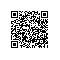# OpenCV+python 人脸识别# 绪论

1992年麻省理工学院通过实验对比了基于结构特征的方法与基于模版匹配的方法，发现模版匹配的方法要优于基于特征的方法。

1.可以快速计算简单矩形特征作为人脸图像特征

# 人脸检测OpenCV中常用的特征分类器有两类：Haar特征和LBP特征1.正例样本，即待检测的目标样本
2.反例样本，即其他任意的图片# -*- coding: utf-8 -*-
"""
Created on Wed Jun 22 20:59:21 2016

"""

# -*- coding: utf-8 -*

import numpy as np
import cv2

gray = cv2.cvtColor(img, cv2.COLOR_BGR2GRAY)
# 识别输入图片中的人脸对象.返回对象的矩形尺寸
# 函数原型detectMultiScale(gray, 1.2,3,CV_HAAR_SCALE_IMAGE,Size(30, 30))
# gray需要识别的图片
# 1.03：表示每次图像尺寸减小的比例
# 5：表示每一个目标至少要被检测到4次才算是真的目标(因为周围的像素和不同的窗口大小都可以检测到人脸)
# CV_HAAR_SCALE_IMAGE表示不是缩放分类器来检测，而是缩放图像，Size(30, 30)为目标的最小最大尺寸
# faces：表示检测到的人脸目标序列
for (x,y,w,h) in faces:
if w+h>200:#//针对这个图片画出最大的外框
img2 = cv2.rectangle(img,(x,y),(x+w,y+h),(255,255,255),4)
roi_gray = gray[y:y+h, x:x+w]
roi_color = img[y:y+h, x:x+w]

cv2.imshow('img',img)
cv2.waitKey(0)
cv2.destroyAllWindows()
cv2.imwrite("head.jpg", img) # 保存图片

# 人脸识别

## 降维## PCA人脸识别算法

PCA人脸识别算法的实现步骤如下：
1.首先把所有的训练图片集的每张图片都转换为行向量的形式
2.计算向量集的PCA子空间，并得到特征值和特征向量及均值
3.将训练集的图片与对应的标签都投影到这个PCA子空间，行程一个投影矩阵
4.导入待识别的图像，并进行向量化，也投影到这个PCA子空间
5.计算PCA投影后的训练集向量与待识别图片投影后向量的距离，并找出最接近的那个

# -*- coding: utf-8 -*-
from numpy import *
import numpy as np
import sys,os
import copy
import cv2
import PIL.Image as Image
import matplotlib.pyplot as plt

class Eigenfaces(object):
def __init__(self):
self.eps = 1.0e-16
self.X = []
self.y = []
self.Mat=[]
self.eig_v = 0
self.eig_vect = 0
self.mu = 0
self.projections = []
self.dist_metric=0
classlabel = 0
for dirname, dirnames, filenames in os.walk(path):
for subdirname in dirnames:
sub_path = os.path.join(dirname, subdirname)
for filename in os.listdir(sub_path):
im = Image.open(os.path.join(sub_path, filename))
im = im.convert("L") #数据转换为long类型
self.X.append(np.asarray(im, dtype=np.uint8))
self.y.append(classlabel)
classlabel += 1
# 将图片变为行向量  # 生成图片矩阵
def genRowMatrix(self):
self.Mat = np.empty((0, self.X.size), dtype=self.X.dtype)
for row in self.X:
self.Mat = np.vstack((self.Mat, np.asarray(row).reshape(1,-1)))
# 计算特征脸
def PCA(self, pc_num =0):
self.genRowMatrix()
[n,d] = shape(self.Mat)
if ( pc_num <= 0) or ( pc_num>n):       pc_num = n
self.mu = self.Mat.mean(axis =0)
self.Mat -= self.mu
if n>d:
XTX = np.dot (self.Mat.T,self.Mat)
[ self.eig_v , self.eig_vect ] = linalg.eigh (XTX)
else :
XTX = np.dot(self.Mat,self.Mat.T)
[ self.eig_v , self.eig_vect ] = linalg.eigh (XTX)
self.eig_vect = np.dot(self.Mat.T, self.eig_vect)
for i in xrange(n):
self.eig_vect[:,i] = self.eig_vect[:,i]/linalg.norm(self.eig_vect[:,i])
idx = np.argsort(-self.eig_v)
self.eig_v = self.eig_v[idx]
self.eig_vect = self.eig_vect[:,idx ]
self.eig_v = self.eig_v[0:pc_num ].copy () # select only pc_num
self.eig_vect = self.eig_vect[:,0:pc_num].copy ()

def compute(self):
self.PCA()
for xi in self.X:
self.projections.append(self.project(xi.reshape(1,-1)))

def distEclud(self, vecA, vecB):  # 欧氏距离
return linalg.norm(vecA-vecB)+self.eps

def cosSim(self, vecA, vecB):    # 夹角余弦
return (dot(vecA,vecB.T)/((linalg.norm(vecA)*linalg.norm(vecB))+self.eps))[0,0]
# 映射
def project(self,XI):
if self.mu is None: return np.dot(XI,self.eig_vect)
return np.dot(XI-self.mu, self.eig_vect)
#预测最接近的特征脸
def predict(self,XI):
minDist = np.finfo('float').max
minClass = -1
Q = self.project(XI.reshape(1,-1))
for i in xrange(len(self.projections)):
dist = self.dist_metric(self.projections[i], Q)
if dist < minDist:
minDist = dist
minClass = self.y[i]
return minClass
# 生成特征脸
def subplot(self,title, images):
fig = plt.figure()
fig.text(.5, .95, title, horizontalalignment='center')
for i in xrange(len(images)):
plt.imshow(asarray(images[i]), cmap="gray")
plt.xticks([]), plt. yticks([]) # 隐藏 X Y 坐标
plt.show()
# 归一化
def normalize(self, X, low, high, dtype=None):
X = np.asarray(X)
minX, maxX = np.min(X), np.max(X)
X = X - float(minX)
X = X / float((maxX - minX))
X = X * (high-low)
X = X + low
if dtype is None:
return np.asarray(X)
return np.asarray(X, dtype=dtype)
'''
# 重构
def reconstruct(self,W, Y, mu=None):
if mu is None:  return np.dot(Y,W.T)
return np.dot(Y, W.T) + mu
# 从外部数据计算投影
def out_project(self,W,XI,mu):
if mu is None:  return np.dot(XI,W)
return np.dot(XI-mu, W)
'''# -*- coding: utf-8 -*-

from numpy import *
import sys,os
from pca import *

sys.setdefaultencoding('utf-8')

ef = Eigenfaces()
ef.dist_metric=ef.distEclud
ef.compute()
E = []
X = mat(zeros((10,10304)))
for i in xrange(16):
X = ef.Mat[i*10:(i+1)*10,:].copy()
# X = ef.normalize(X.mean(axis =0),0,255)
X = X.mean(axis =0)
imgs = X.reshape(112,92)
E.append(imgs)
ef.subplot(title="AT&T Eigen Facedatabase", images=E)  

from numpy import *
import sys,os
from pca import *

sys.setdefaultencoding('utf-8')

ef = Eigenfaces()
ef.dist_metric=ef.distEclud
ef.compute()
# 创建测试集
testImg = ef.X
print "实际值 =", ef.y, "->", "预测值 =",ef.predict(testImg)

# 参考文献使用钉钉扫一扫加入圈子
+ 订阅